# Numerical Integration: Gaussian Methods in 1D

The Gaussian integration, known also as the method of gaussian quadrature, is a numerical approximation of a definite integral of a function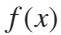in a general interval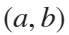. We will apply this approach to the computation of the integral of a polynomial function (which, for sufficiently big n, will give an exact result) and of general functions (approximate result).

Gauss quadrature formula is defined for the reference interval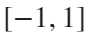as a weighted sume of the values ofat appropiately chosen points: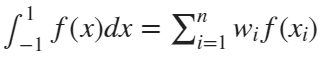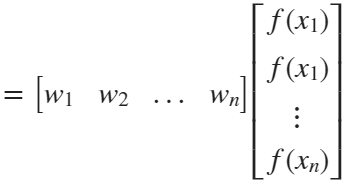.
We need to choose both points and weights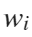. The points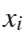are the zeros of Legendre polinomials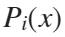. This is a family of polynomial functions represented in the following figure according to the degree (only the first ones). See more information at this Wikipedia link.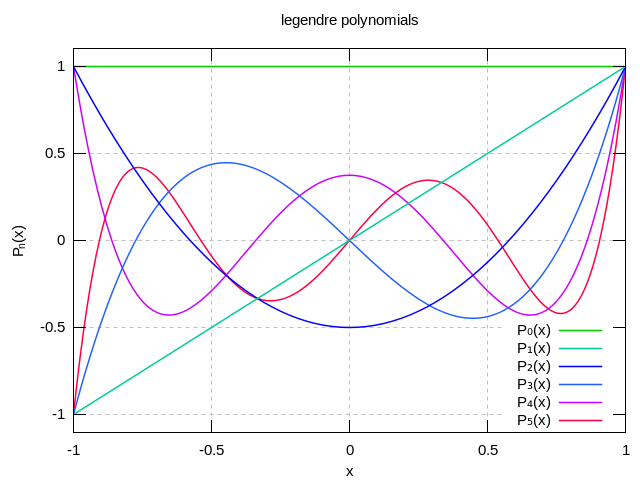For i =1,..,5
The values and weights for these points in the Gauss quadrature formulas are shown in the following table

##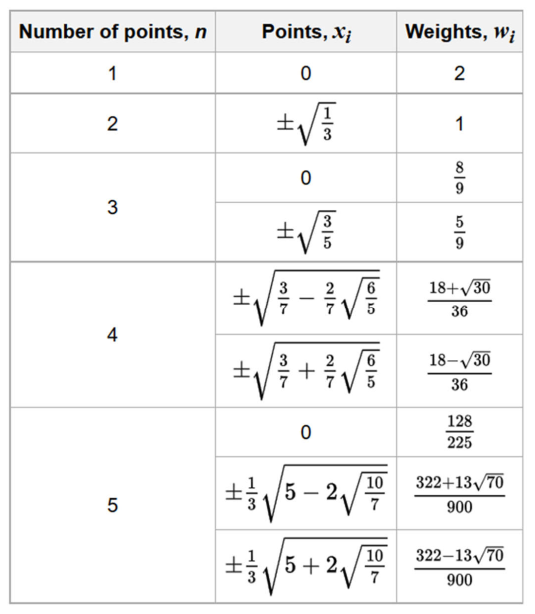For example, if we choose n=3 the Gauss approximation formula is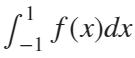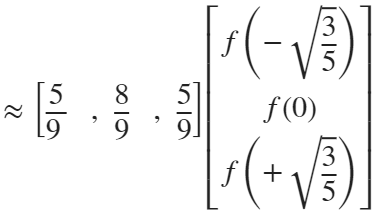.

## Example:

Now we show an example of the different steps needed to compute the Gauss aprimation value of an integral. As you will see, due to thatis a polynomial function, the result is exact when we choose the proper number of Gauss points.

#### Function to integrate

As an example, consider the polynomial function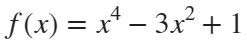, defined in the reference interval [-1,1]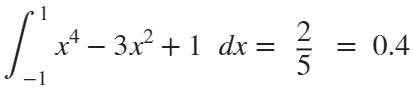First we defined the function as an inline function using Matlab
f=@(x) x.^4-3*x.^2+1; %defined as an inline function

#### Order of integration (number of Gauss points)

When you fix a number of points n, the Gauss integration formula is exact for polynomials of degree 2n-1. In our example the polynomial degree is 4, then if we choose only two points n=2 the integral is still not exact. Therefore, the proper choose is n=3.
n=3;
% because our function is a polynomial of order 4 we have to choose n=3
switch (n) %switch executes only one piece of the code according to the value of n
case 1 %if n=1
w=2; pG=0;
case 2 %if n=2
w=[1,1]; pG=[-1/sqrt(3), 1/sqrt(3)];
case 3 %if n=3
w=[5/9, 8/9, 5/9]; pG=[-sqrt(3/5), 0, sqrt(3/5)];
otherwise
error('No data are defined for this value');
end
fprintf('n=%d , pG = %f %f %f ',n,pG)
n=3 , pG = -0.774597 0.000000 0.774597

#### The final Gauss formula

You can use a sequential formula
sumat = 0;
for i=1:size(pG,2)
sumat = sumat + w(i)*f(pG(i));
end
intFseq = sumat
intFseq = 0.4000
or equivalently, a more compact form of the same procedure
intFcompact = w*f(pG)'
intFcompact = 0.4000

#### Check the error

Here, because it is a simple example, we can compute the primitive of the functionand solve the integral using Barrow's rule. Then, we compare the result with the numerical value coming from the Gauss formula.
primitiveF=@(x) x.^5/5-x.^3+x; %just to check the error
barrowRule=primitiveF(1)-primitiveF(-1);
errorInt = abs(barrowRule - intFcompact)
errorInt = 2.2204e-16

## Generalization to integration over a general interval [a,b]

When the integration interval is not [-1,1] you have to make a change of variables in the intergral in order to apply the previous formulas to the new interval.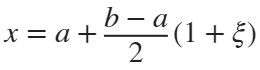being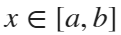and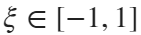.
This means that the Gauss points, initially defined in the interval, must be transformed into points on the new integration interval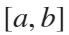#### Example:

Compute the integral of the same function, but now in the interval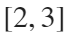. So we have to use the change of variables for integrals.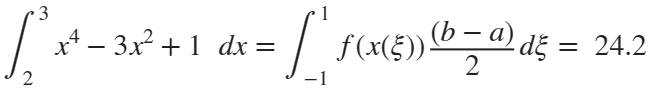a=2;
b=3;
pGnew = a+(b-a)*(1+pG)/2; %we have already the value of pG
intFcompactNew = (w*f(pGnew)')*(b-a)/2 %the weight values are the same
intFcompactNew = 24.2000

## Exercise 1:

According to the table included above, you have to use the function [w,pt] = gaussValues1D(n), included at the end of this script, to see how the approximation of the integral is improved (you must safe the gaussValues1D function in a new file named gaussValues1D.m).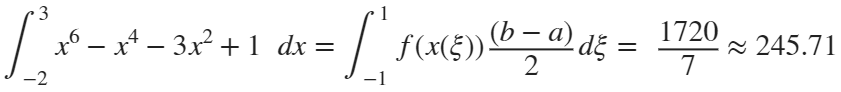f=@(x) x.^6-x.^4-3*x.^2+1; %defined as an inline function
primitiveF=@(x) x.^7/7-x.^5/5-x.^3+x; %just to check the error
barrowRuleNew=primitiveF(3)-primitiveF(-2);
a=-2;
b=3;
for n=1:5 %for n=6 must return an error
[w,pG]=gaussValues1D(n); %function to be implemented
pGnew = a+(b-a)*(1+pG)/2; %we have already the value of pG
intFcompactNew = (w*f(pGnew)')*(b-a)/2; %the weight values are the same
errorInt = abs(barrowRuleNew - intFcompactNew);
fprintf('for n= %d the error is =%e \n',n,errorInt)
end
for n= 1 the error is =2.446987e+02 for n= 2 the error is =1.769180e+02 for n= 3 the error is =2.790179e+01 for n= 4 the error is =2.842171e-13 for n= 5 the error is =4.263256e-13

## Exercise 2:

Using the function gaussValues1D, approximate the value of the integral inof the function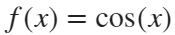. Use different values of n and show the errors comparing with the true integral value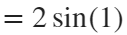Sol:
n, error
1 3.1706e-01
2 7.1183e-03
3 6.1578e-05
4 2.8092e-07
5 7.9140e-10

## Function gaussValues 1D

function [w,pt]=gaussValues1D(n)
switch (n)
case 1
w=2; pt=0;
case 2
w=[1,1]; pt=[-1/sqrt(3), 1/sqrt(3)];
case 3
w=[5/9, 8/9, 5/9]; pt=[-sqrt(3/5), 0, sqrt(3/5)];
case 4
w=[(18+sqrt(30))/36, (18+sqrt(30))/36, (18-sqrt(30))/36, (18-sqrt(30))/36];
pt=[-sqrt(3/7-2/7*(sqrt(6/5))), sqrt(3/7-2/7*(sqrt(6/5))),...
-sqrt(3/7+2/7*(sqrt(6/5))), sqrt(3/7+2/7*(sqrt(6/5)))];
case 5
w=[(322+13*sqrt(70))/900, (322+13*sqrt(70))/900, 128/225,...
(322-13*sqrt(70))/900,(322-13*sqrt(70))/900];
pt=[-1/3*sqrt(5-2*sqrt(10/7)), 1/3*sqrt(5-2*sqrt(10/7)),0,...
-1/3*sqrt(5+2*sqrt(10/7)), 1/3*sqrt(5+2*sqrt(10/7))];
otherwise
error('No data are defined for this value');
end
end
(c) Numerical Factory 2023